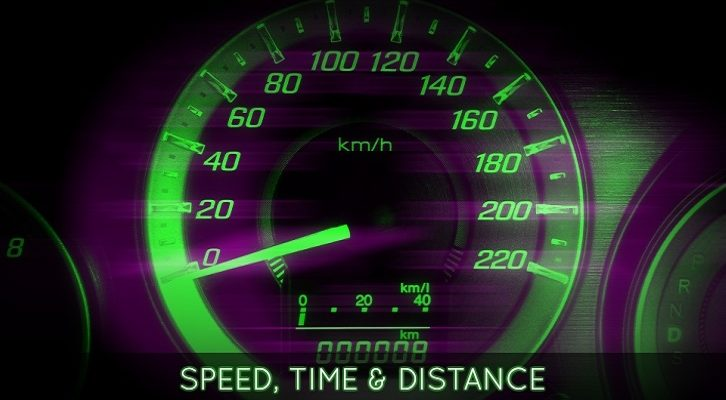# Speed Time Distance – Solutions

Have given below the solutions to the two questions on Speed Time Distance .

1. Two friends A and B leave City P and City Q simultaneously and travel towards Q and P at constant speeds. They meet at a point in between the two cities and then proceed to their respective destinations in 54 minutes and 24 minutes respectively. How long did B take to cover the entire journey between City Q and City P?

Let us assume Car A travels at a speed of a and Car B travels at a speed of b. Further, let us assume that they meet after t minutes.

Distance traveled by car A before meeting car B = a*t. Likewise distance traveled by car B before meeting car A = b * t.

Distance traveled by car A after meeting car B = a *54. Distance traveled by car B after meeting car A = 24* b

Distance traveled by car A after crossing car B = distance traveled by car B before crossing car A (and vice versa)

=> at = 54b ——– 1
and bt = 24a ——– 2

Multiplying equations 1 and 2
we have ab * t2 = 54 * 24 * ab

=> t2 = 54 * 24 => t = 36.

So, both cars would have traveled 36 minutes prior to crossing each other. Or, B would have taken 36 + 24 = 60 minutes to travel the whole distance.

2. Car A trails car B by 50 meters. Car B travels at 45km/hr. Car C travels from the opposite direction at 54km/hr. Car C is at a distance of 220 meters from Car B. If car A decides to overtake Car B before cars B and C cross each other, what is the minimum speed at which car A must travel?

To begin with, let us ignore car A. Car B and car C travel in opposite directions. Their relative speed = Sum of the two speeds = 45 + 54 kmph. = 99kmph. = 99 * 5/18 m/s = 55/2 m/s = 27.5m/s.

The relative distance = 220m. So, time they will take to cross each other = 220/27.5 = 8 seconds.

Now, car A has to overtake car B within 8 seconds. The relative distance = 50m
=> Relative speed should be at least 50/8m/s. = 6.25m/s
= 6.25 * 18/5 kmph = 22.5kmph.

Car B travels at 45kmph, so car A should travel at at least 45 + 22.5 = 67.5kmph.